﻿ Mathematics Formula On Blackboard Stock Image - Image of perimeter, rectangular: 32788041

# Mathematics formula on blackboard.

## Mathematics formula of geometric shape written on blackboard

Photo Taken On: January 31st, 2013

Designers also selected these stock photos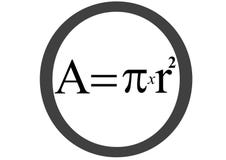Circle area equation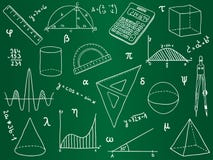Mathematics icons on school board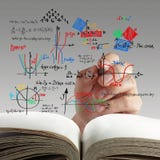Maths and science formula on whiteboard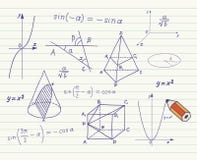Mathematics - geometric shapes and expressions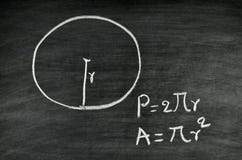Circle area and perimeter formula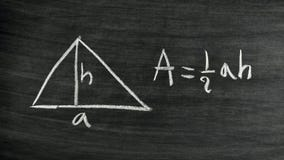Triangle area formula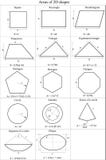Area of 2D shapes - Vector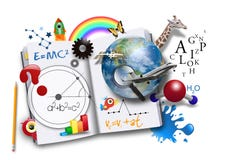Open Learning Book with Science and Math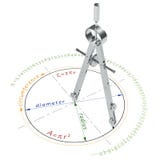Circle and compasses
More similar stock images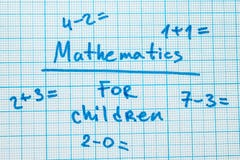The word `mathematics for children` with tasks in a notebook for drawing.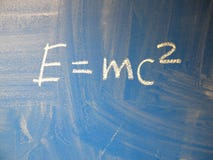Mathematical formula e=mc2 squared written on a blue, relatively dirty chalkboard by chalk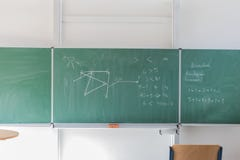Chalkboard with mathematical formulaStudents helps friend teaching and learning subject additional in library. Education concept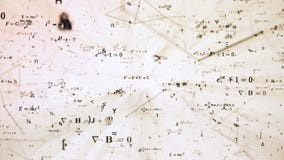Digital futuristic illustration with math, physics formulas in mesh network grid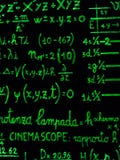Green fluo mathematical calculation on blackboard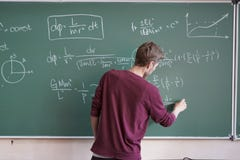Young student in casual writing physics formula on the blackboard during study course s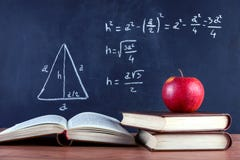Apple on books and blackboard with Pythagorean theorem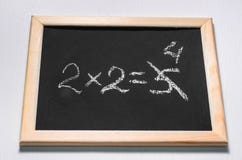A mathematical example
More stock photos from Pupunkkop's portfolio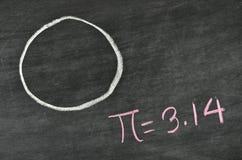Pi number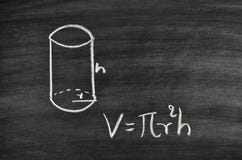Cylindrical volume formula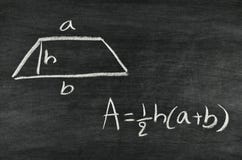Trapezoid area formula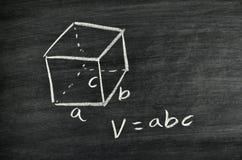Cuboid volume formula
Related categories
Extended licenses